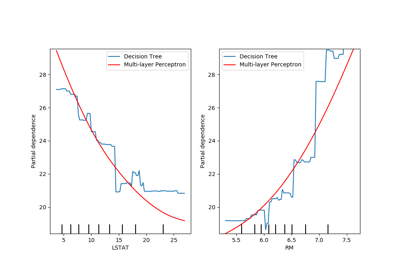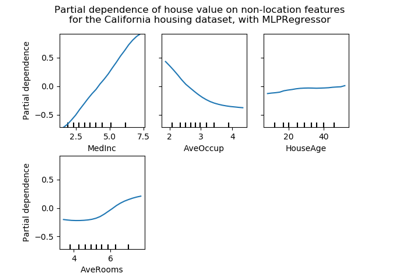# sklearn.inspection.plot_partial_dependence¶

sklearn.inspection.plot_partial_dependence(estimator, X, features, feature_names=None, target=None, response_method='auto', n_cols=3, grid_resolution=100, percentiles=(0.05, 0.95), method='auto', n_jobs=None, verbose=0, fig=None, line_kw=None, contour_kw=None, ax=None)[source]

Partial dependence plots.

The len(features) plots are arranged in a grid with n_cols columns. Two-way partial dependence plots are plotted as contour plots. The deciles of the feature values will be shown with tick marks on the x-axes for one-way plots, and on both axes for two-way plots.

Note

plot_partial_dependence does not support using the same axes with multiple calls. To plot the the partial dependence for multiple estimators, please pass the axes created by the first call to the second call:

>>> from sklearn.inspection import plot_partial_dependence
>>> from sklearn.datasets import make_friedman1
>>> from sklearn.linear_model import LinearRegression
>>> X, y = make_friedman1()
>>> est = LinearRegression().fit(X, y)
>>> disp1 = plot_partial_dependence(est, X)
>>> disp2 = plot_partial_dependence(est, X,
...                                 ax=disp1.axes_)


Read more in the User Guide.

Parameters
estimatorBaseEstimator

A fitted estimator object implementing predict, predict_proba, or decision_function. Multioutput-multiclass classifiers are not supported.

X{array-like or dataframe} of shape (n_samples, n_features)

The data to use to build the grid of values on which the dependence will be evaluated. This is usually the training data.

featureslist of {int, str, pair of int, pair of str}

The target features for which to create the PDPs. If features[i] is an int or a string, a one-way PDP is created; if features[i] is a tuple, a two-way PDP is created. Each tuple must be of size 2. if any entry is a string, then it must be in feature_names.

feature_namesarray-like of shape (n_features,), dtype=str, default=None

Name of each feature; feature_names[i] holds the name of the feature with index i. By default, the name of the feature corresponds to their numerical index for NumPy array and their column name for pandas dataframe.

targetint, optional (default=None)
• In a multiclass setting, specifies the class for which the PDPs should be computed. Note that for binary classification, the positive class (index 1) is always used.

• In a multioutput setting, specifies the task for which the PDPs should be computed.

Ignored in binary classification or classical regression settings.

response_method‘auto’, ‘predict_proba’ or ‘decision_function’, optional (default=’auto’)

Specifies whether to use predict_proba or decision_function as the target response. For regressors this parameter is ignored and the response is always the output of predict. By default, predict_proba is tried first and we revert to decision_function if it doesn’t exist. If method is ‘recursion’, the response is always the output of decision_function.

n_colsint, optional (default=3)

The maximum number of columns in the grid plot. Only active when ax is a single axis or None.

grid_resolutionint, optional (default=100)

The number of equally spaced points on the axes of the plots, for each target feature.

percentilestuple of float, optional (default=(0.05, 0.95))

The lower and upper percentile used to create the extreme values for the PDP axes. Must be in [0, 1].

methodstr, optional (default=’auto’)

The method to use to calculate the partial dependence predictions:

• ‘recursion’ is only supported for gradient boosting estimator (namely GradientBoostingClassifier, GradientBoostingRegressor, HistGradientBoostingClassifier, HistGradientBoostingRegressor) but is more efficient in terms of speed. With this method, X is optional and is only used to build the grid and the partial dependences are computed using the training data. This method does not account for the init predictor of the boosting process, which may lead to incorrect values (see warning below. With this method, the target response of a classifier is always the decision function, not the predicted probabilities.

• ‘brute’ is supported for any estimator, but is more computationally intensive.

• ‘auto’: - ‘recursion’ is used for estimators that supports it. - ‘brute’ is used for all other estimators.

n_jobsint, optional (default=None)

The number of CPUs to use to compute the partial dependences. None means 1 unless in a joblib.parallel_backend context. -1 means using all processors. See Glossary for more details.

verboseint, optional (default=0)

Verbose output during PD computations.

figMatplotlib figure object, optional (default=None)

A figure object onto which the plots will be drawn, after the figure has been cleared. By default, a new one is created.

Deprecated since version 0.22: fig will be removed in 0.24.

line_kwdict, optional

Dict with keywords passed to the matplotlib.pyplot.plot call. For one-way partial dependence plots.

contour_kwdict, optional

Dict with keywords passed to the matplotlib.pyplot.contourf call. For two-way partial dependence plots.

axMatplotlib axes or array-like of Matplotlib axes, default=None
• If a single axis is passed in, it is treated as a bounding axes

and a grid of partial dependence plots will be drawn within these bounds. The n_cols parameter controls the number of columns in the grid.

• If an array-like of axes are passed in, the partial dependence

plots will be drawn directly into these axes.

• If None, a figure and a bounding axes is created and treated

as the single axes case.

New in version 0.22.

Returns
display: PartialDependenceDisplay

Warning

The ‘recursion’ method only works for gradient boosting estimators, and unlike the ‘brute’ method, it does not account for the init predictor of the boosting process. In practice this will produce the same values as ‘brute’ up to a constant offset in the target response, provided that init is a consant estimator (which is the default). However, as soon as init is not a constant estimator, the partial dependence values are incorrect for ‘recursion’. This is not relevant for HistGradientBoostingClassifier and HistGradientBoostingRegressor, which do not have an init parameter.

sklearn.inspection.partial_dependence

Return raw partial dependence values

Examples

>>> from sklearn.datasets import make_friedman1

## Examples using sklearn.inspection.plot_partial_dependence¶